Overview: COUNTREG Procedure

The COUNTREG (count regression) procedure analyzes regression models in which the dependent variable takes nonnegative integer or count values. The dependent variable is usually an event count, which refers to the number of times an event occurs. For example, an event count might represent the number of ship accidents per year for a given fleet. In count regression, the conditional mean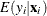of the dependent variableis assumed to be a function of a vector of covariates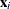.

The Poisson (log-linear) regression model is the most basic model that explicitly takes into account the nonnegative integer-valued aspect of the outcome. With this model, the probability of an event count is determined by a Poisson distribution, where the conditional mean of the distribution is a function of a vector of covariates. However, the basic Poisson regression model is limited because it forces the conditional mean of the outcome to equal the conditional variance. This assumption is often violated in real-life data. Negative binomial regression is an extension of Poisson regression in which the conditional variance can exceed the conditional mean. Also, an often encountered characteristic of count data is that the number of zeros in the sample exceeds the number of zeros predicted by either the Poisson or negative binomial model. Zero-inflated Poisson (ZIP) and zero-inflated negative binomial (ZINB) models explicitly model the production of zero counts to account for excess zeros and also enable the conditional variance of the outcome to differ from the conditional mean.

Under zero-inflated models, additional zeros occur with probability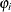, which is determined by a separate model,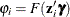, where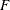is the normal or logistic distribution function that results in a probit or logistic model and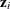is a set of covariates.

PROC COUNTREG supports the following models for count data:

• Poisson regression

• negative binomial regression with quadratic (NEGBIN2) and linear (NEGBIN1) variance functions (Cameron and Trivedi 1986)

• zero-inflated Poisson (ZIP) model (Lambert 1992)

• zero-inflated negative binomial (ZINB) model

In recent years, count data models have been used extensively in economics, political science, and sociology. For example, Hausman, Hall, and Griliches (1984) examine the effects of research and development expenditures on the number of patents received by U.S. companies. Cameron and Trivedi (1986) study factors that affect the number of doctor visits. Greene (1994) studies the number of derogatory reports to a credit reporting agency for a group of credit card applica nts. As a final example, Long (1997) analyzes the number of doctoral publications in the final three years of Ph.D. studies.

The COUNTREG procedure uses maximum likelihood estimation. When a model with a dependent count variable is estimated using linear ordinary least squares (OLS) regression, the count nature of the dependent variable is ignored. This can lead to negative predicted counts and to parameter estimates with undesirable properties in terms of statistical efficiency, consistency, and unbiasedness unless the mean of the counts is high, in which case the Gaussian approximation and linear regression might be satisfactory.